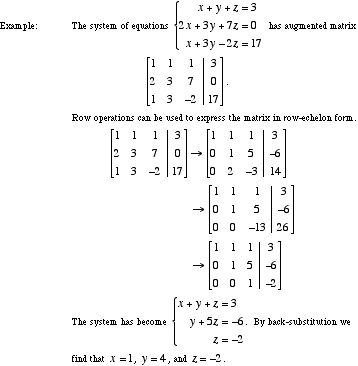index: click on a letter A B C D E F G H I J K L M N O P Q R S T U V W X Y Z A to Z index index: subject areas numbers & symbols sets, logic, proofs geometry algebra trigonometry advanced algebra & pre-calculus calculus advanced topics probability & statistics real world applications multimedia entrieswww.mathwords.com about mathwords website feedback

 Gaussian Elimination A method of solving a linear system of equations. This is done by transforming the system's augmented matrix into row-echelon form by means of row operations. Then the system is solved by back-substitution.See also Gauss-Jordan elimination